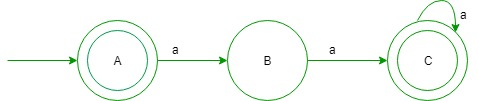# Automata Theory | Set 7

These questions for practice purpose for GATE CS Exam.

Ques-1: Consider L= {(TM) | TM is the Turing machine that halts on all input and L(TM)= L’ for some undecidable language L’}. Here, (TM) is the encoding of a Turing machine as a string over alphabet {0, 1} then L is:

(A) decidable and recursively enumerable
(B) decidable and recursive
(C) decidable and non-recursive
(D) undecidable and recursively enumerable

Explanation:
Here, TM is Turing machine that halts on all input, so L(TM) is decidable and you know that halting Turing machine accepts recursive language and compliment of recursive language can be recursively enumerable which is undecidable.

Hence, L is decidable and recursive. Option (B) is correct.

Ques-2: Which of the following problem(s) is/are decidable?

(A) given two Context sensitive grammar P and Q, is L(P)= L(Q)?
(B) given a Context sensitive grammar P, is L(P) finite or not?
(C) given a Context free grammar P, is L(P) finite or not?
(D) given two deterministic Context free grammar P and Q, is L(P)= L(Q)?

Explanation:
Since, you know that for context free language finiteness problem is decidable.

So, option (C) is correct.

Ques-3: Which of the following statement is true?

(A) given a recursively enumerable grammar P, L(P) is regular language.
(B) given two Context free grammar P and Q, L(P)= L(Q).
(C) given two deterministic Context free grammar P and Q, L(P) is subset of L(Q).
(D) given a Context sensitive grammar P, compliment of L(P) is same as L(P).

Explanation:
Since, you know that for a Context sensitive language compliment of a given language is a language of same type.

So, option (D) is correct.

Ques-4: Consider L= {(ap)* | P is a prime number} over the alphabet {a}, then what is the minimum number of states in NFA that accepts the language L?

(A) three
(B) five
(C) two
(D) four

Explanation:

```L = {(ap)* | P is a prime number}
L = (a2)* U (a3)* U (a5)* U ......
L = {epsilon, a2, a3, a4, a5, ......}  ```

That is, all strings of a’s except ‘a’In the above NFA, only string ‘a’ is not accepted. Hence, number of states required is three.

So, option (A) is correct.

Ques-5: Consider the Context free grammars over the alphabet {a, b} given below, where ‘S’ is non-terminal

```X:S= aSa |aSb |epsilon
Y:S= aaS |bbS |epsilon ```

What is the length of the shortest string which does not belongs to L(X) but belongs to L(Y)?

(A) three
(B) four
(C) one
(D) six

Explanation:
Here, L(X)= set of even palindromes and L(Y)= (aa + bb)*
So, string “aabb” or “bbaa” belongs to L(Y) but does not belongs to L(X). Hence, four is the answer.

Option (B) is true.My Personal Notes arrow_drop_upCheck out this Author's contributed articles.

If you like GeeksforGeeks and would like to contribute, you can also write an article using contribute.geeksforgeeks.org or mail your article to contribute@geeksforgeeks.org. See your article appearing on the GeeksforGeeks main page and help other Geeks.

Please Improve this article if you find anything incorrect by clicking on the "Improve Article" button below.

Improved By : shubham_singh

Article Tags :

Be the First to upvote.

Please write to us at contribute@geeksforgeeks.org to report any issue with the above content.